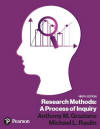﻿ Nonparametric StatisticsGraziano & Raulin
Research Methods (9th edition)

## Nonparametric Statistics

So far, all of the procedures covered in this statistical concepts section of the Student Resource Website have involved estimating population parameters, such as population means and standard deviations. Such tests are called parametric statistics, and they represent by far the most common statistical tests used in psychology.

However, there are times when parametric tests are inappropriate. The most common situation when parametric tests should be avoided is when the scale of measurement for the variables is either nominal or ordinal. There are statistical procedures that are used in those situations that are called nonparametric statistics, because they do not involve estimating population parameters.

We will cover the most commonly used nonparametric procedures in this section and provide references to some of the less commonly used procedures. You can either click on each of these topics in the table of contents below or click on the next page button to move to the next topic.

 Chi Square Statistics Other Nonparametric Statistics